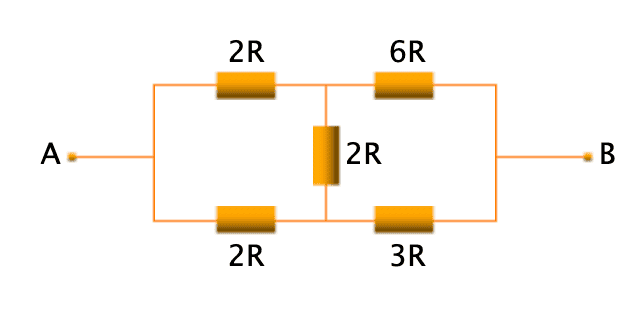# Find equivalent resistance R in the circuit

• valhakla
It can help simplify more complex circuits. Just be careful with the algebra and make sure to include the delta-wye transformation in your final answer. In summary, the discussion covers the concept of parallel resistors and how they must be directly connected to each other with no intervening resistance. The use of various circuit analysis methods is also mentioned, including Kirchhoff's laws and delta-wye transformations. The conversation also offers a helpful resource for further understanding. Ultimately, the delta-wye transformation method is recommended for solving the given problem due to its ability to simplify complex circuits.f

#### valhakla

Homework Statement
Help me to find the total resistance between two points A and B. I have tried to find the total resistance using the formulas Rparralel1 = 2R * 2R /2R+2R, Rparallel2 = 6R *3R / 6R + 3R, and R total = Rparralel1 + Rparralel2 + 2R, the answer was incorrect. Can you tell me where is my mistake, and how can I determine parallel and series resistors to find the total resistance in such circuits?
Relevant Equations
R_parallel = R1 * R2 / R1 + R2, R_series = R1 + R2Rparralel1 = 2R * 2R /2R+2R,
The two R2 on the left are not parallel. To count as parallel, the two resistors must be directly connected to each other, with no intervening resistance, at each end.

I should add to what @haruspex noted that if two resistors are not parallel, this does not mean that they must be in series. It is entirely possible to have circuits where there are no parallel or series combinations.

@valhakla What circuit analysis methods have you learned? Have you covered KCL, KVL? How about mesh and nodal analysis? Perhaps delta-Y and y-delta transforms?

•phinds
@valhakla What circuit analysis methods have you learned? Have you covered KCL, KVL? How about mesh and nodal analysis? Perhaps delta-Y and y-delta transforms
Yes, I have tried to apply Kirchkhoff's and loop rule for this ciruit, but it didn't helped, as it gives only the values of the current flowing through particular resistor not the total resistance between points A and B. Regarding the transforms, I am not familiar with that yet.

The two R2 on the left are not parallel. To count as parallel, the two resistors must be directly connected to each other, with no intervening resistance, at each end.
I understood. So are there any algorithms to approach such circuits? This is my first time encountering this.

•valhakla
Yes, I have tried to apply Kirchkhoff's and loop rule for this ciruit
That’s enough to solve the problem. If that’s all you have covered in class so far, I’d guess that’s what you are meant to use.

Add a battery (emf ##= E##) between A and B so you have a complete circuit.

Analyse the circuit using Kirchhoff’s first law (the ‘current rule’) and second law (the ‘loop rule’). You can find the total current, ##I##, entering A (or leaving B) in terms of ##E## and ##R##.

The resistance between A and B is then ##\frac EI##. ##E## should cancel and the answer will be some multiple of ##R##.

It’s messy because you have a lot of unknown currents.

Note the circuit is ‘linear’. Without loss of generality, you can consider the case with ##R=1 \Omega## and take the battery’s emf as some convenient arbitrary value (e.g. ##E =10V##). The final answer will be a multiple of ##R##, not ohms. However, if you are not comfortable with this ‘shortcut’ you will need to work with the symbols ##R## and ##E##.

Post your working if you need further help.

Also, note spelling: Kirchhoff.

•DaveE
It helped, thank you!

Last edited:
Add a battery (emf ##= E##) between A and B so you have a complete circuit.
I tried to add 10V battery, but my final answer wasn't even close. However the delta-wye transformation method helped me to finally solve the problem.

I tried to add 10V battery, but my final answer wasn't even close.
The risk of algebra/arithmetic errors is high with 5 unknowns! But it will work.

However the delta-wye transformation method helped me to finally solve the problem.
It's a good choice if you are allowed to use that method.

•valhakla